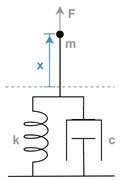Model a System Algorithm

When incomplete system requirements and a developing system design prevent you from defining file management and model architecture upfront, you can still model fundamental system algorithms. By organizing the model into inputs, outputs, and systems, you create a general framework for model components as the model grows.

To show the first stage of a modeling workflow that begins with limited information, this example uses a simple mechanical system composed of a mass, spring, and damper.This second-order differential equation characterizes the system:where

•is mass

•is the damping coefficient

•is the spring constant

•is acceleration

•is velocity

•is displacement

•is force

Solving forprovides a form of this equation that maps more clearly to a Simulink® model.In model ex_modeling_simple_system, a Sum block computes the forces applied to the mass, the Gain block labeled 1/m computes the acceleration of the mass, and the Second-Order Integrator block solves for the velocity and position of the mass.These blocks, which represent the system, are grouped in an area. The two other areas contain system inputs and outputs. By organizing the model upfront, you create a general framework for model components as the model grows.

Since this example shows a model in the preliminary stages of development, the actual input force is unknown and can be represented by a variety of standard source blocks. Model ex_modeling_simple_system uses a Step block connected as input to the system. Some alternative source blocks are shown, but commented out. For example, you can use the From Spreadsheet block to load empirical data if it were available.

Similarly, a variety of sink blocks can accept the output displacement. To check whether simulation results meet expectations, model ex_modeling_simple_system uses a Scope block to visualize the signals.